Students can download 10th Science Chapter 4 Electricity Questions and Answers, Notes, Samacheer Kalvi 10th Science Guide Pdf helps you to revise the complete Tamilnadu State Board New Syllabus, helps students complete homework assignments and to score high marks in board exams.

## Tamilnadu Samacheer Kalvi 10th Science Solutions Chapter 4 Electricity

### Samacheer Kalvi 10th Science Electricity Text Book Back Questions and Answers

Question 1.
Which of the following is correct?
(a) Rate of change of charge is electrical power.
(b) Rate of change of charge is current.
(c) Rate of change of energy is current.
(d) Rate of change of current is charge.
(b) Rate of change of charge is current.

Question 2.
SI unit of resistance is:
(a) mho
(b) joule
(c) ohm
(d) ohm meter
(c) ohmQuestion 3.
In a simple circuit, why does the bulb glow when you close the switch?
(a) The switch produces electricity.
(b) Closing the switch completes the circuit.
(c) Closing the switch breaks the circuit.
(d) The bulb is getting charged.
(b) Closing the switch completes the circuit

Question 4.
Kilowatt hour is the unit of:
(a) resistivity
(b) conductivity
(c) electrical energy
(d) electrical power
(c) electrical energy

II. Fill in the blanks.

1. When a circuit is open, ……….. cannot pass through it.
2. The ratio of the potential difference to the current is known as ……….
3. The wiring in a house consists of ………… circuits.
4. The power of an electric device is a product of ……… and ………..
5. LED stands for ………..

1. current
2. resistance
3. parallel
4. potential difference, current
5. Light Emitting Diode

III. State whether the following statements are true or false: If false correct the statement.

1. Ohm’s law states the relationship between power and voltage.
2. MCB is used to protect house hold electrical appliances.
3. The SI unit for electric current is the coulomb.
4. One unit of electrical energy consumed is equal to 1000 kilowatt hour.
5. The effective resistance of three resistors connected in series is lesser than the lowest of the individual resistances.

1. False – Ohm’s law states that the relationship between current and voltage.
2. True
3. False – The SI unit for electric current is ampere.
4. False – One unit of electrical energy consumed is equal to 1 kilowatt hour.
5. False – The effective resistance of three resistors connected in series is greater than the highest of the “individual resistance.

IV. Match the items in column-1 to the items in column-ll.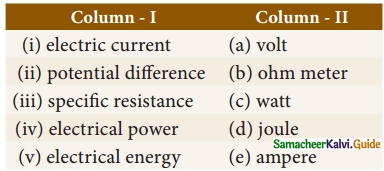(i) – (e)
(ii) – (a)
(iii) – (b)
(iv) – (c)
(v) – (d)

V. Assertion and reason type Questions.

Mark the correct choice as
(a) If both the assertion and the reason are true and the reason is the correct explanation of the assertion.
(b) If both the assertion and the reason are true, but the reason is not the correct explanation of the assertion.
(c) If the assertion is true, but the reason is false.
(d) If the assertion is false, but the reason is true.
1. Assertion: Electric appliances with a metallic body have three wire connections.
Reason: Three pin connections reduce heating of the connecting wires.
(b) If both the assertion and the reason are true, but the reason is not the correct explanation of the assertion.

2. Assertion: In a simple battery circuit the point of highest potential is the positive terminal of the battery.
Reason: The current flows towards the point of the highest potential.
(b) If both the assertion and the reason are true, but the reason is not the correct explanation of the assertion.

3. Assertion: LED bulbs are far better than incandescent bulbs.
Reason: LED bulbs consume less power than incandescent bulbs.
(a) If both the assertion and the reason are true and the reason is the correct explanation of the assertion.Question 1.
Define the unit of current.
The current flowing through a conductor is said to be one ampere, when a charge of one coulomb flows across any cross-section of a conductor, in one second. Hence,
1 ampere = $$\frac{1 coulomb}{1 second}$$

Question 2.
What happens to the resistance, as the conductor is made thicker?
If the conductor is made a thicker area of cross-section of conduction increases that will decrease the resistance.

Question 3.
Why is tungsten metal used in bulbs, but not in fuse wires?
Tungsten metal is used in bulbs because its melting point is the greatest.
But it is not used in fuse wires. When a current more than 5A flows tungsten wire will be melted. Hence tungsten is not used in fuse wire.

Question 4.
Name any two devices, which are working on the heating effect of the electric current.
The heating effect of electric current is used in many home appliances such as electric iron and electric toaster.

Question 1.
Define electric potential and potential difference.
Electric Potential: It is the amount of work done in moving unit positive charge from infinity to that point against the electric force.
Electric potential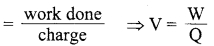Potential difference : It is the amount of work done in moving a unit positive charge from one point to another against the electric force.
Potential difference VA – VB = $$\frac{W_{A}-W_{B}}{Q}$$

Question 2.
What is the role of the earth wire in domestic circuits?

1. The earth wire provides a low resistance path to the electric current.
2. The earth wire sends the current from the body of the appliance to the Earth, whenever a live wire accidentally touches the body of the metallic electric appliance.
3. Thus, the earth wire serves as a protective conductor, which saves us from electric shocks.

Question 3.
State Ohm’s law.
According to Ohm’s law, at a constant temperature, the steady current ‘I’ flowing through a conductor is directly proportional to the potential difference ‘V’ between the two ends of the conductor.
I ∝ V. Hence, = $$\frac{1}{V}$$ = constant.
The value of this proportionality constant is found to $$\frac{1}{R}$$
Therefore, I = ($$\frac{1}{R}$$) V
V = IR

Question 4.
Distinguish between the resistivity and conductivity of a conductor.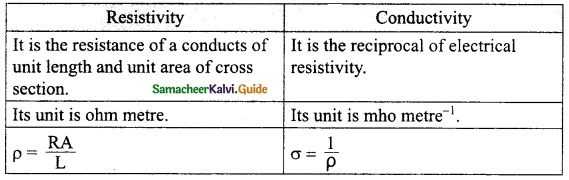Question 5.
What connection is used in domestic appliances and why?

1. In the domestic appliance, it is used as a parallel connection to avoid short circuit and breakage.
2. It has an alternative current (AC). Not DC current as it is from cables, so high potential flows through this.
3. One more advantage of the parallel connection of circuits is that each electric appliance gets an equal voltage.Question 1.
With the help of a circuit diagram derive the formula for the resultant resistance of three resistances connected:
(a) in series and
(b) in parallel
(a) Resistors in series : A series circuit connects the components one after the other to form a ‘single loop’. A series circuit has only one loop through which current can pass. If the circuit is interrupted at any point in the loop, no current can pass through the circuit and hence no electric appliances connected in the circuit will work. Series circuits are commonly used in devices such as flashlights. Thus, if resistors are connected end to end, so that the same current passes through each of them, then they are said to be connected in series.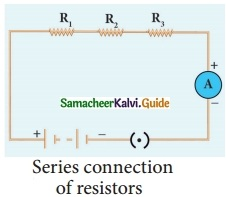Let, three resistances R1, R2 and R3 be connected in series. Let the current flowing through theorem be I. According to Ohm’s Law, the potential differences V1,V2 and V3 across R1, R2 and R3 respectively, are given by:
V1 = I R1 ………. (1)
V2 = I R2 ……… (2)
v3 = I R3 ………. (3)
The sum of the potential differences across the ends of each resistor is given by:
V = V1 + V2 + V3
Using equations (1), (2) and (3), we get
V = I R1 + I R2 + I R3 …….. (4)
The effective resistor is a single resistor, which can replace the resistors effectively, so as to allow the same current through the electric circuit. Let, the effective resistance of the series-combination of the resistors, be RS.
Then,
V = I RS ……….(5)
Combining equations (4) and (5), we get,
I RS = I R1 + I R2 + I R3
RS = R1 + R2 + R3 ……….. (6)
Thus, you can understand that when a number of resistors are connected in series, their equivalent resistance or effective resistance is equal to the sum of the individual resistances. When ‘n’ resistors of equal resistance R are connected in series, the equivalent resistance is ‘n R’.
i.e., RS = n R
The equivalent resistance in a series combination is greater than the highest of the individual resistances.

(b) Resistors in Parallel : A parallel circuit has two or more loops through which current can pass. If the circuit is disconnected in one of the loops, the current can still pass through the other loop(s). The wiring in a house consists of parallel circuits.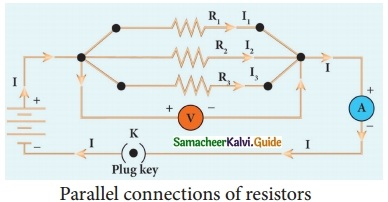Consider that three resistors R1, R2 and R3 are connected across two common points A and B The potential difference across each resistance is the same and equal to the potential difference between A and B. This is me sured using the voltmeter. The current I arriving at A divides into three branches I1, I2 and I3 passing through R1, R2 and R3 respectively.
According to the Ohm’s law, you have,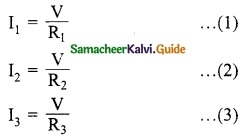The total current through the circuit is given by
I = I1 + I2 + I3
Using equations (1), (2) and (3), you get
I = $$\frac{V}{R_1}$$ + $$\frac{V}{R_2}$$ + $$\frac{V}{R_3}$$ ……… (4)
Let the effective resistance of the parallel combination of resistors be RP Then,
I = $$\frac{V}{R_P}$$ ……… (5)
Combining equations (4) and (5), you have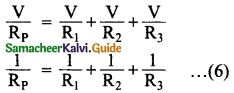Thus, when a number of resistors are connected in parallel, the sum of the reciprocals of the individual resistances is equal to the reciprocal of the effective or equivalent resistance. When ‘n’ resistors of equal resistances R are connected in parallel, the equivalent resistance is $$\frac{R}{n}$$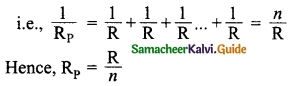The equivalent resistance in a parallel combination is less than the lowest of the individual resistances.

Question 2.
(a) What is meant by electric current? Give its direction?
(b) Name and define its unit.
(c) Which instrument is used to measure the electric current? How should it be r connected in a circuit?
(a) (i) Electric current is often termed as ‘current’ and it is represented by the symbol ‘I’. It is defined as the rate of flow of charges in a conductor.
(ii) The electric current represents the number of charges flowing in any cross-section of a conductor (say a metal wire) in unit time.

(b) The SI unit of electric current is ampere (A).
The current flowing through a conductor is said to be one ampere, when a charge of one coulomb flows across any cross-section of a conductor, in one second. Hence,
1 ampere = $$\frac{1 \text { coulomb }}{1 \text { second }}$$

(c) (i) The ammeter is used to measure the current.
(ii) An Ammeter is connected in series with the circuit.
(iii) The Ammeter is a low impedance device connecting it in parallel with the circuit would cause a short circuit, damaging the Ammeter or the circuit.

Question 3.
(a) State Joule’s law of heating.
(b) An alloy of nickel and chromium is used as the heating element. Why?
(c) How does a fuse wire protect electrical appliances?
(a) Joule’s law of heating states that the heat produced in any resistor is:

1. directly proportional to the square of the current passing through the resistor.
2. directly proportional to the resistance of the resistor.
3. directly proportional to the time for which the current is passing through the resistor.

(b) Because,

1. It has high resistivity,
2. It has a high melting point,
3. It is not easily oxidized.

(c) When a large current passes through the circuit, the fuse wire melts due to Joule’s heating effect and hence the circuit gets disconnected. Therefore, the circuit and the electric appliances are saved from any damage.Question 4.
Explain about domestic electric circuits, (circuit diagram not required)

1. Electricity is distributed through the domestic electric circuits wired by the electricians.
2. The first stage of the domestic circuit is to bring the power supply to the main-box from a distribution panel, such as a transformer.
3. The important components of the main-box are: (i) a fuse box and (ii) a meter. The meter is used to record the consumption of electrical energy.
4. The fuse box contains either a fuse wire or a miniature circuit breaker (MCB).
5. The function of the fuse wire or an MCB is to protect the household electrical appliances from overloading due to excess current.

Question 5.
(a) What are the advantages of LED TV over the normal TV?
(b) List the merits of LED bulb.

1. It has brighter picture quality.
2. It is thinner in size.
3. It uses less power and consumes very less energy.
4. Its life span is more.
5. It is more reliable.

1. As there is no filament, there is no loss of energy in the form of heat.
2. It is cooler than the incandescent bulb.
3. In comparison with the fluorescent light, the LED bulbs have significantly low power requirement.
4. It is not harmful to the environment.
5. A wide range of colours is possible here.
6. It is cost-efficient and energy efficient.
7. Mercury and other toxic materials are not required. One way of overcoming the energy crisis is to use more LED bulbs.

IX. Numerical problems.

Question 1.
An electric iron consumes energy at the rate of 420 W when heating is at the maximum rate and 180 W when heating is at the minimum rate. The applied voltage is 220 V. What is the current in each case?
(i) When heating is maximum, the power
P1 = 420 W
Applied voltage V = 220 V
P = VI
Current I = $$\frac{P}{V}$$
I = $$\frac{420}{220}$$ = 1.909 A
I = 1.909 A

(ii) When heating is minimum
Power P2 = 180 W
Applied voltage V = 220 V
P = VI
∴ Current I = $$\frac{P}{V}$$
I = $$\frac{180}{220}$$ = 0.8181 A
I = 0.8181 A

Question 2.
A 100-watt electric bulb is used for 5 hours daily and four 60 watt bulbs are used for 5 hours daily. Calculate the energy consumed (in kWh) in the month of January.
Solution:
100 W = 100 joules per second
1 watt hours = 3600 joules
The electric bulb is lighted for 5 hours daily,
100 W × 5 = 500 watt hours
500 watt hours = 1800000 joules
1 kWh = 3600000 joules
Units consumed per day = $$\frac{1800000}{3600000}$$ = 0.5 units
Untis consumed in month = 0.5 × 31 = 15.5 units …. (1)
Now, Sum of power of four 60 watt bulbs = 240 W
240 W × 5 hours = 1200 watt hours
1200 watt hours = 4320000 joules
Energy consumed per day = $$\frac{4320000}{3600000}$$ = 1.2 units
Energy consumed in a month = 1.2 × 31 = 37.2 units …. (2)
Total energy consumed in a month = 15.5 + 37.2 = 52.7 units
1 unit = 1 kWh
The energy consumed in the month of January = 52.7 kWh.

Question 3.
A torch bulb is rated at 3 V and 600 mA. Calculate it’s
(a) power
(b) resistance
(c) energy consumed if it is used for 4 hour.
Voltage V = 3 V
Current I = 600 mA
(a) Power = VI
= 3 × 600 × 10-3
= 1800 × 10-3
= 1.8 W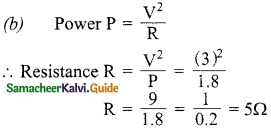(c) Time = 4h
Energy consumed E = P × t
E = 1.8 × 4
= 7.2 WQuestion 4.
A piece of wire having a resistance R is cut into five equal parts.
(a) How will the resistance of each part of the wire change compared with the original resistance?
(b) If the five parts of the wire are placed in parallel, how will the resistance of the combination change?
(c) What will be ratio of the effective resistance in series connection to that of the parallel connection?
(a) Original resistance
R = $$\frac{l}{A}$$
∴ R α l
After cutting length of each piece
r = $$\frac{l}{5}$$
New resistance
R’ = $$\frac{l’}{A}$$
R’ α l’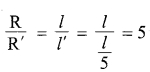R : R’ = 5 : 1

(b) When five parts of the wire are placed in parallel.
Effective Resistance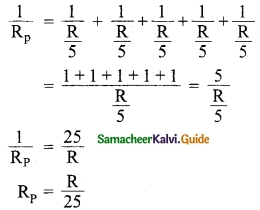Resistance of the combinations
RP = $$\frac{R}{25}$$

(c) When resistance are connected in series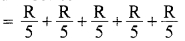RS = R
When resistance are connected in parallel
RP = $$\frac{R}{25}$$
RS : RP = R : $$\frac{R}{25}$$
= 25 R : R_1
RS : RP = 25 : 1

X. HOT Questions.

Question 1.
Two resistors when connected in parallel give the resultant resistance of 2 ohm; but when connected in series the effective resistance becomes 9 ohm. Calculate the value of each resistance.
Let the resistance be R, and R2 when two resistances are connected in series
RS = R1 + R2
= 9
R1 + R2 = 9 ……….(1)
When two resistance are connected in parallel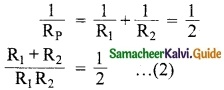Using (1) equation (2) becomes
$$\frac{9}{R_{1}R_2}$$
R1 R2 = 18 ……….(3)
(R1 – R2)² = (R1 + R2)² – 4R1R2
= (9)² – 4 × 18
= 81 – 72 = 9
∴ (R1 – R2) = √9 = 3 ………(4)
From (1)
R1 + R2 = 9
2R1 = 12
∴ R1 = $$\frac{12}{2}$$ = 6 ohm
From (1)
R2 = 9 – R1
= 9 – 6 = 3Ω
The values of resistances are
R1 = 6 ohm
R2 = 3 ohm

Question 2.
How many electrons are passing per second in a circuit in which there is a current of 5 A?
Solution:
Current I = 5A
Time (t) = 1 second
Charge of electron e = 1.6 × 10-19 C
$$\mathbf{I}=\frac{q}{t}=\frac{n e}{t}$$
Number of electron, n = $$\frac{\mathrm{I} t}{e}$$ = $$\frac{5 \times 1}{1.6 \times 10^{-19}}$$
n = 3.125 × 1019.

Question 3.
A piece of wire of resistance 10 ohm is drawn out so that its length is increased to three times its original length. Calculate the new resistance.
Resistance R = 10Ω
Let l be the length of the wire R ∝ 1
When the length is increased to three times,
l’ = 3l
∴ New Resistance
R’ ∝ l’ ∝ 3l
∴ $$\frac{R}{R’}$$ = $$\frac{l}{3l}$$ = $$\frac{1}{3}$$
∴ R’ = 3R
New resistance = 3 times the original resistance.Question 1.
Electric current is defined as the rate of flow of:
(a) energy
(b) power
(c) mass
(d) charge
(d) charge

Question 2.
The S.I. unit of electric current is _____.
(a) Volt
(b) Power
(c) Ampere
(d) newton.
(c) Ampere

Question 3.
The unit of electric current is:
(a) ampere
(b) volt
(c) watt
(d) kilo-watt
(a) ampere

Question 4.
The work done in moving a charge of 2 C across two points in a circuit is 2 J. What is the potential difference between the points?
(a) 1 V
(b) 10 V
(c) 100 V
(d) 0.
(a) 1 V

Question 5.
The amount of work done to move a unit charge from one point to the other is:
(a) resistance
(b) current
(c) Potential
(d) none of the above
(c) Potential

Question 6.
Ohm’s law gives the relative between potential difference and:
(a) emf
(b) temperature
(c) resistance
(d) current
(d) current

Question 7.
The unit of resistance is _____.
(a) volt
(b) volt ampere-1
(c) ampere
(d) Joule.
(b) volt ampere-1

Question 8.
The symbol of battery is: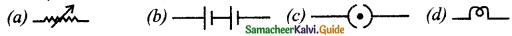(b)

Question 9.
Electrical resistivity for a given material is ______.
(a) zero
(b) constant
(c) both (a) and (b)
(d) only (b).
(b) constantQuestion 10.
The potential difference required to pass a current 0.2 A in a wire of resistance 20 ohm is:
(a) 100 V
(b) 4 V
(c) 0.01 V
(d) 40 V
(b) 4 V

Question 11.
The unit of electrical conductivity ______.
(a) ohm-1 metre
(b) ohm-1 metre-1
(c) Volt Ampere-1
(d) ohm.
(b) ohm-1 metre-1

Question 12.
Kilowatt-hour is the unit of:
(a) potential difference
(b) electric power
(c) electric energy
(d) charge
(c) electric energy

Question 13.
The resistivity of a material is 4 × 10-8 Ωm and its conductivity ______.
(a) 25 × 10-8 mho m-1
(b) 0.25 × 10-8 mho m-1
(c) 25 × 108 mho m-1
(d) 0.25 × 108 mho m-1.
(d) 0.25 × 108 mho m-1.

Question 14.
The commonly used safely fuse wire is made of:
(a) nickel
(c) an alloy of tin and lead
(d) copper
(c) an alloy of tin and lead

Question 15.
The value of one horse power is:
(a) 746 kW
(b) 746 W
(c) 3.6 × 105 W
(d) 3.6 × 106 kW
(b) 746 W

Question 16.
When ‘n’ number of resistors are connected in series, the effecive resistance for series is ______.
(a) nR
(b) $$\frac{n}{\mathrm{R}}$$
(c) $$\frac{\mathbf{R}}{n}$$
(d) none of these.
(a) nR

Question 17.
Name the physical quantity which is measured in kW:
(a) electric energy
(b) electric power
(c) electric current
(d) electric potential
(b) electric power

Question 18.
What is the amount of current, when 20 C of charges flows in 4 s through a conductor? [l = $$\frac{q}{v}$$]
(a) 5 A
(b) 80 A
(c) 4 A
(d) 2 A
(a) 5 A

Question 19.
Nichrome is ______.
(a) a conductor
(b) an insulator
(c) an alloy
(d) none of these.
(c) an alloy

Question 20.
The main source of biomass energy is:
(a) coal
(b) heat energy
(c) thermal energy
(d) cow-dung
(d) cow-dung

Question 21.
The value of one ampere is:
(a) $$\frac{1second}{1coulomb}$$
(b) 1 coulomb × sec
(c) $$\frac{1coulomb}{1second}$$
(d) 1 coulomb
(c) $$\frac{1coulomb}{1second}$$

Question 22.
The heat produced in an electric heater of resistance 2 Ω is connected to an electric source, when a current of 6 A flows for 5 minutes _____.
(a) 216 × 102 J
(b) 2160 J
(c) 900 J
(d) 150 J.
(a) 216 × 102 J
Hint: Formula H = I2Rt Joule.

Question 23.
The value of $$\frac{1joule}{1coulomb}$$
(a) 1 kWh
(b) 1 Wh
(c) ampere
(d) volt
(d) voltQuestion 24.
The mathematical from of Ohm’s law is given by:
(a) V = IR
(b) I = VR
(c) R = $$\frac{I}{V}$$
(d) I = $$\frac{R}{V}$$
(a) V = IR

Question 25.
One kilowatt hour is _____.
(a) 3.6 × 106 J
(b) 36 × 106 J
(c) 3.6 × 105 J
(d) 36 × 105 J.
(a) 3.6 × 106 J

Question 26.
If the length and radius of a conductor is doubled then its specific resistance will:
(a) be doubled
(b) be halved
(c) be tripled
(d) remain the same
(d) remain the same

Question 27.
The value of resistivity of nichrome is:
(a) 1.5 × 106 Ωm
(b) 1.5 × 10-6 Ωm
(c) 5.1 × 106 Ωm
(d) 5.1 × 10-6 Ωm
(b) 1.5 × 10-6 Ωm

Question 28.
Due to short circuit, effective resistance in the circuit becomes _____.
(a) large
(b) very small
(c) very large
(d) zero.
(b) very small

Question 29.
if a conductor has a length of 1 m and area of 1 m² then its resistivity is equal to its:
(a) resistance
(b) conductance
(c) length
(d) conductivity
(a) resistance

Question 30.
When resistors are connected in parallel, potential difference across each resistor will be:
(a) different
(b) same
(c) vary
(d) none of the above
(b) same

Question 31.
LED TV screen was developed by James P. Mitchell in _____.
(a) 1797
(b) 1977
(c) 2009
(d) 1987.
(b) 1977

Question 32.
Heat developed across a conductor H =
(a) IRt
(b) VR
(c) I2Rt
(d) I2R
(c) I2Rt

Question 33.
Expression for electric energy is:
(a) W = $$\frac{V}{I}$$
(b) W = VIt
(c) W = Vt
(d) W = $$\frac{V}{It}$$
(b) W = VIt

Question 34.
In our home, fuse box consists of:
(a) fuse wire
(b) MCB
(c) fuse wire or MCB
(d) switches
(c) fuse wire or MCB

Question 35.
Which of the following is a semi conductor device?
(a) LED bulb
(b) fuse
(c) MCB
(d) switch
(a) LED bulbII. Fill in the blanks.

1. The flow of charge: Electric current. A continuous closed path of an electric current is ………. The unit of charge: Coulomb then-current ……….
2. Electric current I: Charge (Q)/ ………. while electric potential V is ……….
3. A resistor of resistance R: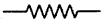Then variable resistance and ……….. rheostat ……….
4. In series connection of resistors: ………. Then for parallel connection of resistors: ………..
5. The transformation of energy in Electric oven: ………… Electric cell ………..
6. The expression obtained from Ohm’s law ………… joule’s law
7. The unit of electric power …………. then electric energy ………..
8. The equivalent of 1 volt ………. then for 1 ohm ………..
9. The tap-key is used to ……… and ……….. an electric circuit.
10. The opposition to flow of current is called ………. and its unit is ………..
11. The heat developed in a conductor is directly proportional to the square of ………… and ………. of flow.
12. The S.I unit of electric current is ……….
13. The S.l unit of resistance is ……….
14. ……….. is the S.l unit of potential difference.
15. From Ohm’s law $$\frac{V}{I}$$ =
16. If a current 2A flows through conductor having a potential difference of 6 V then its resistance is ……….
17. If R is the resistance of a conductor then its conductance is G = ……….
18. Conductivity is ……… for ……….. than insulators.
19. When resistors are connected in series the equivalent resistance is …………. than the highest resistance of individual resistors.
20. In series connection ……….. is less as effective resistance is more.
21. Tungsten is used as heating element because its resistance is ……….
22. Tungsten is used as filament in the electric bulb because its melting points is …………
23. If a current of 6A flows through a 5Ω resistance for 10 minutes than heat developed in the resistance is ……….
24. When a current of IA flows through a conductor having potential difference of IV, the electric power is ………..
25. 746 watt is equivalent to ………..
26. In displays are used ………….
1. Electric circuit, Ampere
2. Time (t), Work done (W)/charge (Q)
3.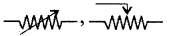4. Current is same, Potential difference is same
5. electrical into heat energy, chemical into electrical energy
6. V = IR, H = I2Rt
7. Kilowatt, want hour
8. $$\frac{1joule}{1coulomb}$$, $$\frac{1volt}{1ampere}$$
9. open, close
10. resistance, ohm
11. current, time
12. ampere
13. Ohm 15. R
14. Volt
15. R
16. 3 ohm
17. $$\frac{I}{R}$$
18. more, conductors
19. greater
20. Current
21. high
22. high
23. 108 kJ
24. 1 W
25. 1 horse power
26. LED bulbs

III. State whether the following statements are true or false: If false correct the statement.

1. Current is the rate of flow of charges
2. The symbol of diode is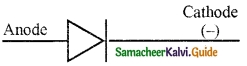3. Potential = $$\frac{charge}{time}$$
4. Mathematical form of ohm’s law is V = IR
5. Nichrome is used in electric bulb.
6. The unit of conductance is mho.
7. The equivalent resistance in a parallel combination is less than the lowest of the individual resistance.
8. In parallel connection the effective resistance is RP = $$\frac{R_{1}+R_{2}}{R_{1}R_{2}}$$
9. Heat produced in a conductor is H = l²Rt
10. 1 kWh = 3.6 J.
11. An MCB is a switching device.
12. LED means Light Emitting Diode.
1. True
2. True
3. False – Potential = $$\frac{Workdone(W)}{Charge(Q)}$$
4. True
5. False – Nichrome is used in heating device.
6. True
7. True
8. False – In parallel connection, the effective resistance is RP = $$\frac{R_{1}R_{2}}{R_{1}+R_{2}}$$
9. True
10. False – 1 kWh = 3.6 × 106 J
11. True
12. TrueIV. Match the items in column-1 to the items in column-ll.

Question 1.
Match the Column I with Column II.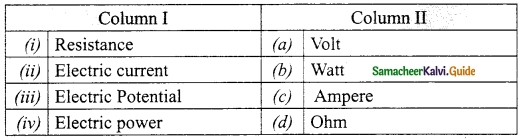(i) – (d)
(ii) – (c)
(iii) – (a)
(iv) – (b)

Question 2.
Match the components with symbols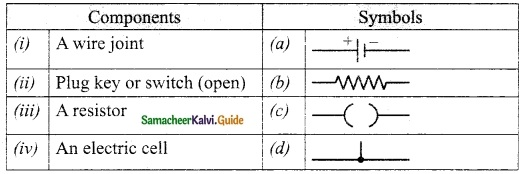(i) – (d)
(ii) – (c)
(iii) – (b)
(iv) – (a)

Question 3.
Match the Column I with Column II.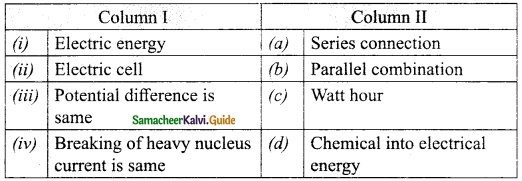(i) – (c)
(ii) – (d)
(iii) – (b)
(iv) – (a)

Question 4.
Match the Column I with Column II.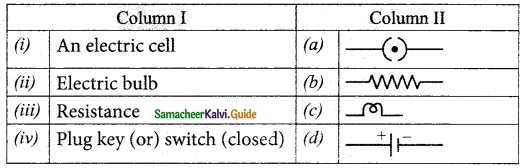(i) – (d)
(ii) – (c)
(iii) – (b)
(iv) – (a)

Question 5.
Match the column I with column II.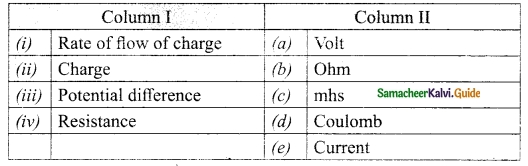(i) – (e)
(ii) – (d)
(iii) – (a)
(iv) – (b)

Question 6.
Match the column I with column II: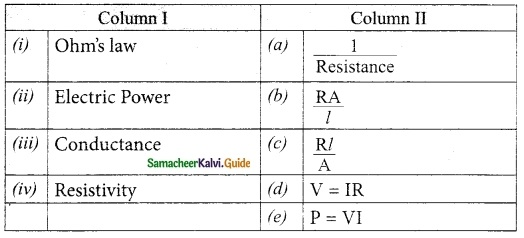(i) – (d)
(ii) – (e)
(iii) – (a)
(iv) – (b)

Question 7.
Match the column I with column II: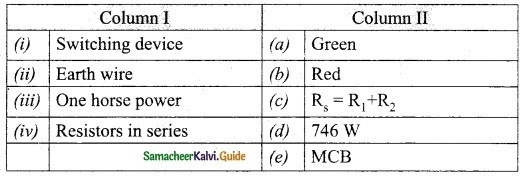(i) – (e)
(ii) – (a)
(iii) – (d)
(iv) – (c)V. Assertion and reason type questions.

Question 1.
Assertion: In a series system, equivalent resistance is the sum of the individual resistance.
Reason: The current that passes through each resistor is the same.
(a) Assertion is true but Reason is false.
(b) Assertion is true and Reason doesn’t explains Assertion,
(c) Both Assertion and Reason are false.
(d) Assertion is true and Reason explains Assertion
(d) Assertion is true and Reason explains Assertion

Question 2.
Assertion: In a parallel system, the total current is equal to the sum of the current through each resistor.
Reason: The potential difference across each resistor is the same.
(a) Assertion is true and Reason explains Assertion.
(b) Assertion is true and Reason doesn’t explains Assertion.
(c) Both Assertion and Reason are false
(d) Assertion is true but Reason is false.
(a) Assertion is true and Reason explains Assertion.

Question 3.
Assertion: The unit of power watt is not frequently used in practice. Reason: it cannot be converted into Joule.
(a) Both Assertion and Reason are false.
(b) Assertion is true but Reason is false.
(c) Both Assertion and Reason are true and Reason explains Assertion.
(d) Both Assertion and Reason are true and Reason doesn’t explains Assertion.
(b) Assertion is true but Reason is false.

Question 4.
Assertion: A wire carrying a current has electric field around d.
Reason: A wire carrying current is stays electrically neutral.
(a) If both the assertion and the reason a re true and the reason is the correct explanation of the assertion.
(b) If both the assertion and the reason are true, but the reason is not the correct explanation of the assertion.
(c) If the assertion is true, but the reason is false.
(d) If the assertion is false, but the reason is true.
(b) If both the assertion and the reason are true, but the reason is not the correct explanation of the assertion.

Question 5.
Assertion: In order to pass current through electric circuit, it must be closed.
Reason: In our home, the switch is ON, then the current flows through the bulb. So, the bulb glows.
(a) If both the assertion and the reason are true and the reason is the correct explanation of the assertion.
(b) If both the assertion and the reason are true, but the reason is not the correct explanation of the assertion.
(c) If the assertion is true, but the reason is false.
(d) If the assertion is false, but the reason is true.
(a) If both the assertion and the reason are true and the reason is the correct explanation of the assertion.

Question 6.
Assertion: Resistance of a material opposes the flow of charges.
Reason: It is different for different materials.
(a) If both the assertion and the reason are true and the reason is the correct explanation of the assertion.
(b) If both the assertion and the reason are true, but the reason is not the correct explanation of the assertion.
(c) If the assertion is true, but the reason is false.
(d) If the assertion is false, but the reason is true.
(b) If both the assertion and the reason are true, but the reason is not the correct explanation of the assertion.

Question 7.
Assertion: Electrical conductivity is the reciprocal of electrical resistivity. Reason: The unit of conductivity is Ohm.
(a) If both the assertion and the reason are true and the reason is the correct explanation of the assertion.
(b) If both the assertion and the reason are true, but the reason is not the correct explanation of the assertion.
(c) If the assertion is true, but the reason is false.
(d) If the assertion is false, but the reason is true.
(c) If the assertion is true, but the reason is false.

Question 8.
Assertion: One end of the earthing wire is connected to a body of the electrical appliance and its other end is connected to a metal tube that is burried into the Earth.
Reason: The earth wire provides low resistance path to the electric current.
(a) If both the assertion and the reason are true and the reason is the correct explanation of the assertion.
(b) If both the assertion and the reason are true, but the reason is not the correct explanation of the assertion.
(c) If the assertion is true, but the reason is false.
(d) If the assertion is false, but the reason is true.
(a) If both the assertion and the reason are true and the reason is the correct explanation of the assertion.Question 9.
Assertion: The passage of electric current through a wire results in the production of heat.
Reason: The heating effect is used in electric heater electric iron etc.
(a) If both the assertion and the reason are true and the reason is the correct explanation of the assertion.
(b) If both the assertion and the reason are true, but the reason is not the correct explanation of the assertion.
(c) If the assertion is true, but the reason is false.
(d) If the assertion is false, but the reason is true.
(b) If both the assertion and the reason are true, but the reason is not the correct explanation of the assertion.

Question 10.
Assertion: One kilowatt hour is known as one unit of electrical energy.
Reason: 1 kWh = 3.6 × 106J
(a) If both the assertion and the reason are true and the reason is the correct explanation of the assertion.
(b) If both the assertion and the reason are true, but the reason is not the correct explanation of the assertion.
(c) If the assertion is true, but the reason is false.
(d) If the assertion is false, but the reason is true.
(a) If both the assertion and the reason are true and the reason is the correct explanation of the assertion.

VI. Very short answer type Questions

Question 1.
If a charge of QC flows through a conductor in time t second then what is the value of current?
Charge = $$\frac{Charge}{time}$$
I = $$\frac{Q}{t}$$

Question 2.
What is the electric circuit?
An electric circuit is a closed conducting loop.
(or)
path, which has a network of electrical components through which electrons are able to flow. This path is made using electrical wires so as to connect an electric appliance to a source of electric charges (battery).

Question 3.
If the length of a wire is doubled and its cross-section is also doubled than what happens its resistance?
Resistance of a wire
R = $$\frac{pl}{A}$$ ………. (1)
l’ = 2l
A’ = 2A
∴ New Resistance
R’ = l × $$\frac{2l}{2A}$$
R’ = $$\frac{pl}{A}$$ …….. (2)
Form (1) and (2) it is found that resistance remains unchanged.

Question 4.
What is the unit of resistance and resistivity of a conductor?
(i) The unit of resistance is ohm.
(ii) The unit of resistivity is ohm meter.

Question 5.
Define the unit of resistance? (or) Define one ohm.
The resistance of a conductor is said to be one ohm if a current of one ampere flows through it when a potential difference of one volt is maintained across its ends.
$$\text { Ohm }=\frac{1 \text { volt }}{1 \text { ampere }}$$.

Question 6.
What is the unit of conductivity?
The unit of conductivity is mho meter.Question 7.
What is the value of one kilo watt hour?
One kilowatt hour = 1000 W hr
1 kWh = 3.6 × 106J

Question 8.
What is the heating effect of electric current?
The passage of electric current through a wire resulting in the production of heat. This phenomenon is called the heating effect of current. This heating effect of current is used in devices like electric heater, electric iron, etc.

Question 1.
What is an electric circuit?
An electric circuit is a closed conducting loop (or) path, which has a network of electrical components through which electrons are able to flow.

Question 2.
Draw a circuit diagram to represent a simple electric circuit.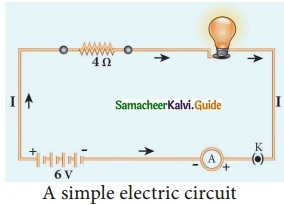Question 3.
What is the direction of conventional current?
By convention, the direction of current is taken as the direction of flow of positive charge (or) opposite to the direction of flow of electrons.

Question 4.
Define electric potential.
The electric potential at a point is defined as the amount of work done in moving a unit positive charge from infinity to that point against the electric force.

Question 5.
(i) Overloading happens when a large number of appliances are connected in series to the same source of electric power. This leads to a flow of excess current in the electric circuit.

(ii) When the amount of current passing through a wire exceeds the maximum permissible limit, the wires get heated to such an extent that a fire may be caused. This is known as overloading.

Question 6.
What is meany by short circuit?

1. When a live wire comes in contact with a neutral wire, it causes a ‘short circuit’.
2. This happens when the insulation of the wires get damaged due to temperature changes or some external force.
3. Due to a short circuit, the effective resistance in the circuit becomes very small, which leads to the flow of a large current through the wires.
4. This results in heating of wires to such an extent that a fire may be caused in the building.

Question 7.
Draw an electric circuit to understand Ohm’s law.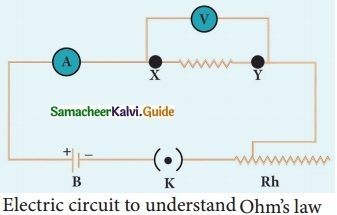Question 8.
Define resistance of a conductor.
The resistance of a conductor can be defined as the ratio between the potential difference across the ends of the conductor and the current flowing through it.

Question 9.
Define Resistance. Give its unit and conductance.
The resistance of a conductor can be defined as the ratio between the potential difference across the ends of the conductor and the current flowing through it.
Conductance: It is defined as the reciprocal of its resistance (R). Hence, the conductance ‘G’ of a conductor is given by
$$\mathrm{G}=\frac{1}{\mathrm{R}}$$
Its unit is ohm-1. It is also represented as ‘mho’.

Question 10.
Define electrical resistivity of a material.
The electrical resistivity of a material is defined as the resistance of a conductor of unit length and unit area of cross section. Its unit is ohm metre.Question 11.
What is meant by electrical conductivity?
The reciprocal of electrical resistivity of a material is called its electrical conductivity.
σ = $$\frac{1}{p}$$

Question 12.
Mention the differences between the combination of resistances in series and parallel.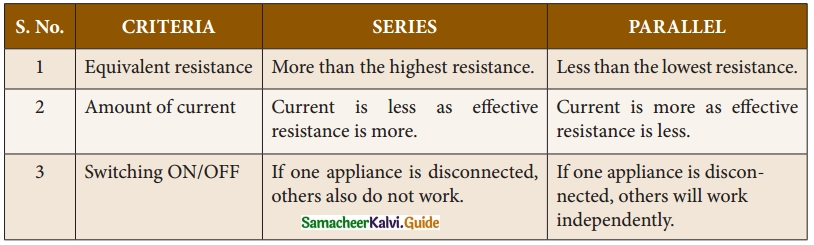Question 13.
Write short notes about filament in electric bulbs.
In electric bulbs, a small wire is used, known as filament. The filament is made up of a material whose melting point is very high. When current passes through this wire, heat is produced in the filament. When the filament is heated, it glows and gives out light. Tungsten is the commonly used material to make the filament in bulbs.

Question 14.
What is meant by electric power?
The electric power is the product of the electric current and the potential difference due to which the current passes in a circuit.

Question 15.
When the amount of current passing through a wire exceeds the maximum permissible limit, the wires get heated to such an extent that a fire may be caused. This is known as overloading.

Question 16.
What is meant by LED bulb?
An LED bulb is a semiconductor device that emits visible light when an electric current passes through it. The colour of the emitted light will depend on the type of materials used.

Question 17.
W hat is meant by seven segment display? state its uses.
(i) A ‘Seven Segment Display’ is the display device used to give an output in the form of numbers or text.
(ii) It is used in digital meters, digital clocks, microwave ovens, etc.

Question 18.
What do you know about LED television.
LED Television is one of the most important applications of Light Emitting Diodes. An LED TV is actually an LCD TV (Liquid Crystal Display) with LED display. An LED display uses LEDs for backlight and an array of LEDs act as pixels. LEDs emitting white light are used in monochrome (black and white) TV; Red, Green and Blue (RGB) LEDs are used in colour television.

Question 19.
What is fuse wire?
The function of fuse wire or an MCB is to protect the household electrical appliances from excess current due to overloading or a short circuit.

Question 20.
Draw a group between potential difference and current.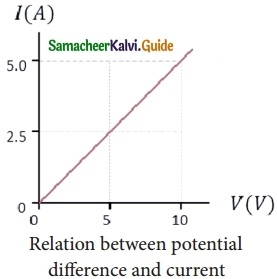Question 21.
Write short note about short circuit?
When a live wire comes in contact with a neutral wire, it causes a ‘short ‘ circuit’. This happens when the insulation of the wires get damaged due to temperature changes or some external force. Due to a short circuit, the effective resistance in the circuit becomes very small, which leads to the flow of a large current through the wires. This results in heating of wires to such an extent that a fire may be caused in the building.

Question 1.
Tabulate various components used in electrical circuit and their uses?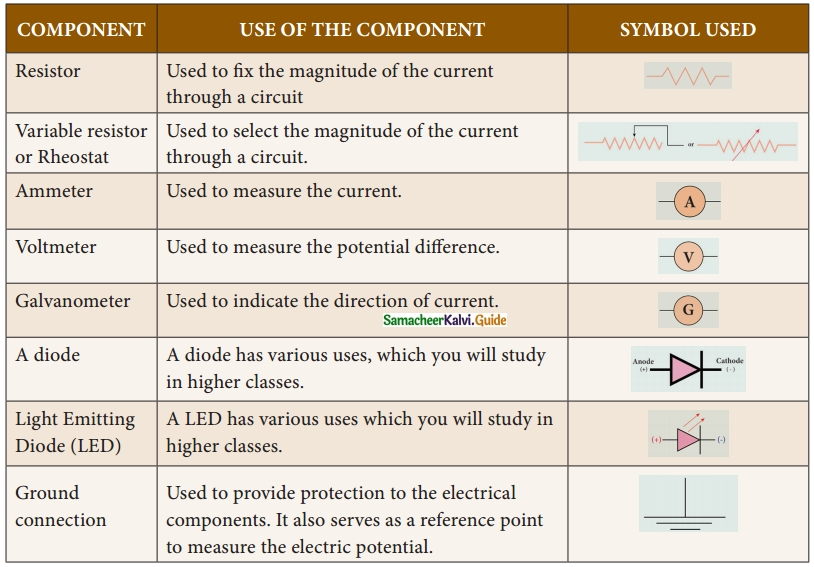Question 2.
Explain series connection of parallel resistors.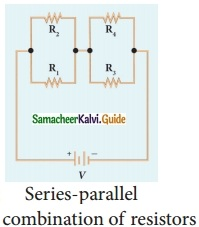If we consider the connection of a set of parallel resistors that are connected in series, you get a series – parallel circuit. Let R1 and R2 be connected in parallel to give an effective resistance of Rp1. Similarly, let R3, and R4 be connected in parallel to give an effective resistance of Rp2. Then, both of these parallel segments are connected in series. Using equation we get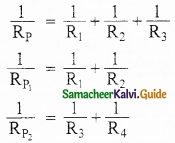We get = R1 + R2 + R3 ……….(1)
Finally, using equation (1), the net effective resistance is given by
Rtotal = RP1 + RP2

Question 3.
Explain parallel connection of series resistors.
If you consider a connection of a set of series resistors connected in a parallel circuit, you get a parallel-series circuit. Let R1 and R2 be connected in series to give an effective resistance of RS1. Similarly, let R3 and R4 be connected in series to give an effective resistance of RS2. Then, both of these serial segments are connected in parallel.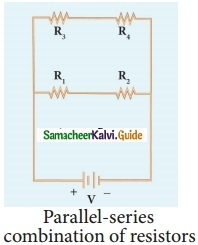Using equation
RS = R1 + R2 + R3
We get RS1 = R1 + R2, RS2 = R3 + R4
Finally, using equation
$$\frac{1}{R_P}$$ = $$\frac{1}{R_1}$$ + $$\frac{1}{R_2}$$ + $$\frac{1}{R_3}$$
the net effective resistance is given by
$$\frac{1}{R_{total}}$$ = $$\frac{1}{R_{S1}}$$ + $$\frac{1}{R_{S2}}$$

Question 4.
Explain applications of heating effect.
Electric Heating Device: The heating effect of electric current is used in many home appliances such as electric iron, electric toaster, electric oven, electric heater, geyser, etc. In these appliances Nichrome, which is an alloy of Nickel and Chromium is used as the heating element. Because:
(i) it has high resistivity,
(ii) it has a highmelting point,
(Hi) it is not easily oxidized.

Fuse Wire : The fuse wire is connected in series, in an electric circuit. When a large current passes through the circuit, the fuse wire melts due to Joule’s heating effect and hence the circuit gets disconnected. Therefore, the circuit and the electric appliances are saved from any damage. The fuse wire is made up of a material whose melting point is relatively low.

Filament in bulbs : In electric bulbs, a small wire is used, known as filament. The filament is made up of a material whose melting point is very high. When current passes through this wire, heat is produced in the filament. When the filament is heated, it glows and gives out light. Tungsten is the commonly used material to make the filament in bulbs.Question 5.
(i) LED bulb
(ii) Seven segment display
(i) An LED bulb is a semiconductor device that emits visible light when an electric current passes through it. The colour of the emitted light will depend on the type of materials used. With the help of the chemical compounds like Gallium Arsenide and Gallium Phosphide, the manufacturer can produce LED bulbs that radiates red, green, yellow and orange colours. Displays in digital watches and calculators, traffic signals, street lights, decorative lights, etc., are some examples for the use of LEDs.

(ii) A ‘Seven Segment Display’ is the display device used to give an output in the form of numbers or text. It is used in digital meters, digital clocks, micro wave ovens, etc. It consists of 7 segments of LEDs in the form of the digit 8. These seven LEDs are named as a, b, c, d, e, f and g. An extra 8th LED is used to display a dot.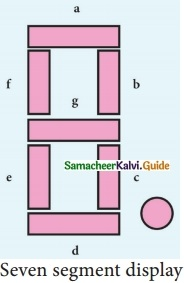IX. Numerical problems.

Question 1.
An electric iron draws a current of 0.5 A when the voltage is 220 volts. Calculate the amount of electric charge flowing through it in one hour.
I = $$\frac{Q}{t}$$
Charge Q = ?
Charge I = 0.5 A
Time = 1 hour
= 60 × 60 s
Q = It
= 0.5 × 3600
= 1800 C
The amount of electric charge flowing = 1800 C

Question 2.
A current of 5A flows through a heater for 10 minutes. Calculate the amount of electric charge flowing through the electric circuit.
Q = I × t
Current I = 5A
Time t = 10 × 60 = 600 s
= 5 × 600 = 3000 C

Question 3.
A torch bulb draws a current 0.6 A, when glowing from a source of 6 V. Calculate the resistance of the bulb when glowing.
V = IR
Current I = 0.6A
Potential V = 6V
R = ?
R = $$\frac{V}{I}$$ = $$\frac{6}{0.6}$$ = 10Ω
The resistance of the bulb = 10Ω

Question 4.
Find the potential difference required to pass a current of 0.2 A in a wire of resistant 20Ω.
V = IR
Current I = 0.2A
Resistance R = 20Ω
Potential difference V = ?
V = 0.2 × 20 = 4V
The potential difference in a wire = 4V

Question 5.
Calculate the amount of work done in moving charge of 25 C across two points having potential difference of 20V.
W = QV
Charge Q = 25 C
Potential difference V = 20 V
W = 25 × 20 = 500 J

Question 6.
Three resistances are connected in an electrical circuit as shown in the circuit diagram. Determine the potential difference across resistance R2.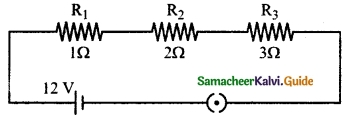For series connection, the effective resistance
RS = R1 + R2 + R3
= 1 + 2 + 3 = 6Ω
Total potential = 12V
Amount of current flowing through the circuit
I = $$\frac{V}{R_{S}}$$ = $$\frac{12V}{6Ω}$$ = 2A
Potential difference across resistance R2
V = I × R2
= 2 × 2 = 4V
The potential difference across the resistance R2 = 4 V

Question 7.
In the given network, find the equivalent resistance between A and B.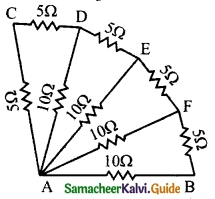An equivalent of the given network is drawn in the relevant parts as follows: Resistance of the combination R1 and R2 is
RS = 5 + 5 = 10Ω
Resistance of the combination R1, R2 and R3 is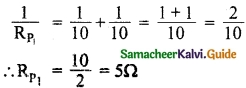The resistance of series combination RP1 and R4 is RS1 = 5 + 5 = 10Ω
Resistance of the combination RS1 and R5 is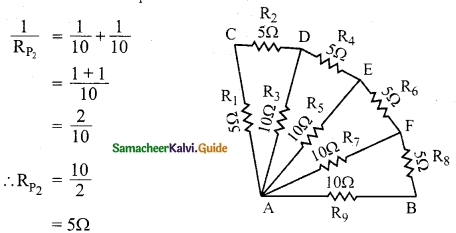Resistance of the series combination RP2 and R6 is
RS2 = 5 + 5 = 10Ω
Resistance of the combination RS2 and R7 is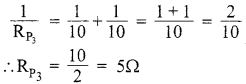Resistance of the series combination RP3 and R8 is
RP3 = 5 + 5 = 10Ω
Resistance of the combination RS3 and R9 is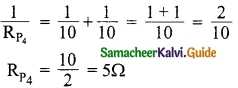Resistance of the series combination RP4 and R6 is
RS4 = 5 + 5 = 10Ω
Resistance of the combination RS4 and R8 is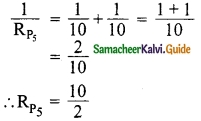∴ Resistance between A and B is 5ΩQuestion 8.
For a given circuit calculate
(i) the total effective resistance of the circuit.
(ii) the total current in the circuit
(iii) the current through each resistor.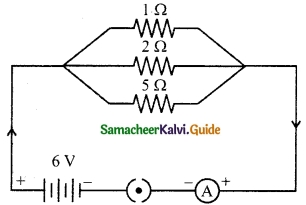For parallel connection, the effective resistance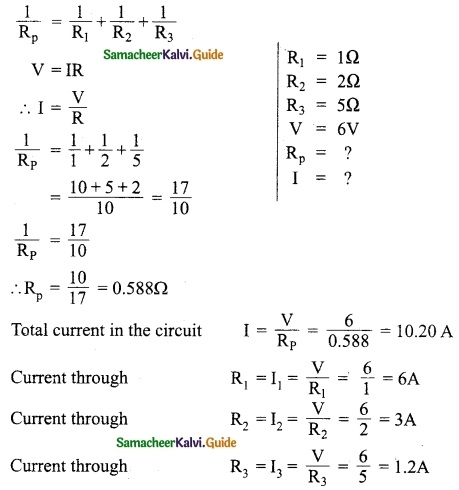(i) Total effective resistance of the circuit
RP = 0.588Ω

(ii) Total current in the circuit
I = 10.2 A

(iii) Current through R1 = 6A
Current through R2 = 3A
Current through R3 = 1.2A

Question 9.
An electric iron a rating of 750 W, 220 V.
(i) Calculate current passing through it and
(ii) Its resistance when in use.
P = VI
I = $$\frac{P}{V}$$
Ohm’s law V = IR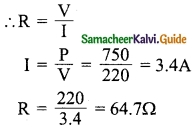(i) The amount of current passing
I = 3.4A
(ii) Resistance
R = 64.7Ω

Question 10.
Following graph was plotted between V and I values. What would be the values of $$\frac{V}{I}$$ ratios when the potential difference is 0.8 V and 1.2 V?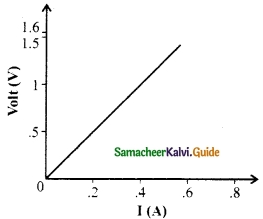V1 = 0.8V, I1 = 32A; V2 = 1.2V; I2 = 48A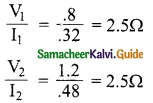Question 11.
Three resistors of 2Ω, 4Ω and 8Ω are connected in parallel with a battery of 3 V. Calculate
(i) Current through each resistor and
(ii) Total current in the circuit.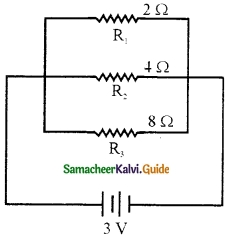Potential difference across each resistor is same.
(i) Current through each resistor: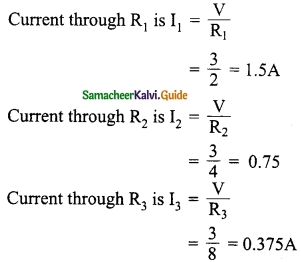(ii) Total current in the circuit: I = I1 + I2 + I3
I = 1.5 + 0.75 + 0. 375
I = 2.625 AQuestion 12.
Two bulbs of 40 W and 60 W are connected in series to an external potential difference. Which bulb will glow brighter? Why?
Let the external potential difference be 230 V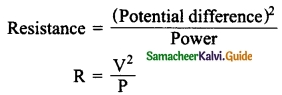For 40 W bulb resistance is R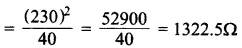For 60 W bulb resistance is R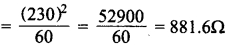According to Ohm’s law
I = $$\frac{V}{R}$$
Current flowing through 40 W bulb is
$$\frac{230}{1322.5}$$ = 0.1739 A
Current flowing through 60 W bulb is
$$\frac{230}{881.6}$$ = 0.2608 A
When bulbs are connected in series effective resistance is
RS = R1+ R2 = 1322.5 + 881.6
RS = 2204.1Ω
Net current
I = $$\frac{230}{2204.1}$$ = 0.1043 A
Using power equation P = I²R
For 40 W bulb P = I²R
= (0.1043)² × 1322.5
= (0.01087) × 1322.5 = 14.386 W
For 60 W bulb P = I²R
= (0.1043)² × 881.6
= (0.01087) × 881.6 = 9.5904 W
In a series system, higher the resistance, higher the brightness so, 40 W bulb glows brighter.

Question 13.
A wire is bent into a circle. The effective resistance across the diameter is 8Ω. Find the resistant if the wire.
RP = 8Ω = resistance across diameter
$$\frac{1}{R}$$ + $$\frac{1}{R}$$ = $$\frac{1}{8}$$
$$\frac{2}{R}$$ = $$\frac{1}{8}$$
R = 16Ω
x is the resistance of the wire.
x = 16 + 16 = 32Ω
x = 32ΩX. HOT Questions.

Question 1.
A 60 W bulb is connected in parallel with a room heater. This combination is connected across the mains. If 60 W bulb is replaced by a 100 W bulb what happens to the heat produced by the heater? Given reason.
Heat produced by the heater will be same. When the bulb and a heater are connected in parallel and this combination is connected across the mains, potential difference across each is the same equal to the voltage V of the mains irrespective of the resistance of the bulb.

If R is the resistance of the heater then heat produced by the heater will be $$\frac{V^2}{R}$$ in both cases. Hence heat produces by heater will not be changed.

Question 2.
Two bulbs 60 W and 100 W are connected in series and this combination is connected to a d.c power supply. Will the potential difference across 60 W bulb be higher than that across 100 W bulb?
60 W bulb has a higher resistance than the resistance across 100 W bulb since the power developed is P = $$\frac{V^2}{R}$$.
Potential difference across a bulb will be proportional to resistance. Hence potential difference across 60 W bulb is higher than that across 100 W bulb.

Question 3.
Super conductors has lowest resistance. Is it true. Give reason.
True. When the temperature of super conductor is reduced to zero or near by zero its resistance becomes zero.

Question 4.
A constant voltage is applied between two ends of a uniform conducting wire. If both the length and radius of the wire is doubled then what happens to the heat produced in the wire?
We know that resistance of a conducting wire is R = $$\frac{pl}{A}$$
$$\frac{Pl}{πr^{2}}$$
If length l and radius r are doubled, then resistance will become half. But heat produced H = $$\frac{V^2}{R}$$.
Hence, heat produced per second will become thrice.

Question 5.
Calculate the effective resistance between A and B.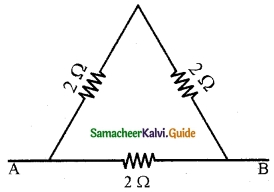The electrical circuit can be redrawn as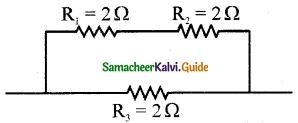The resistance R1 and R2 are in series
RS = R1 + R2 = 2 + 2 = 4Ω
The resistance RS and R3 are in parallel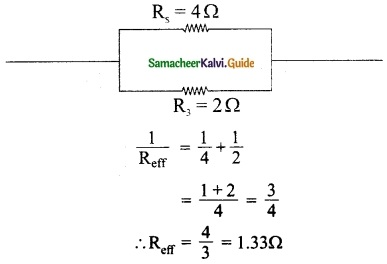∴ Effective resistance Reff= 1.33Ω

Question 6.
Two wires of same material and length have resistances 5Ω and 10Ω respectively. Calculate the ratio of radii of the two wires.
Resistance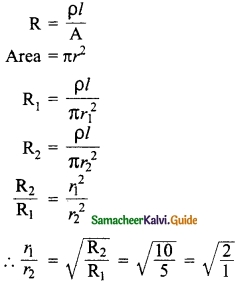∴ r1 : r2 = √2 : 1Question 7.
An iron box of 400 W power is used daily for 30 minutes. If the cost per unit is 75 paise, find the weekly expense on using the iron box.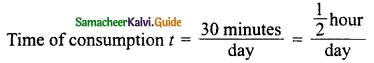= 400 × $$\frac{1}{2}$$ = 200 Wh